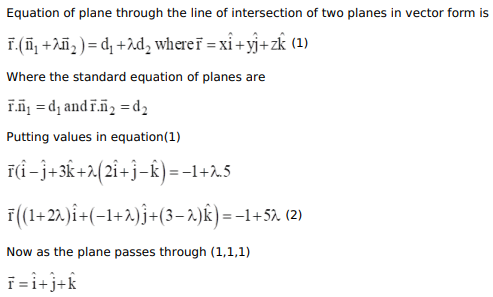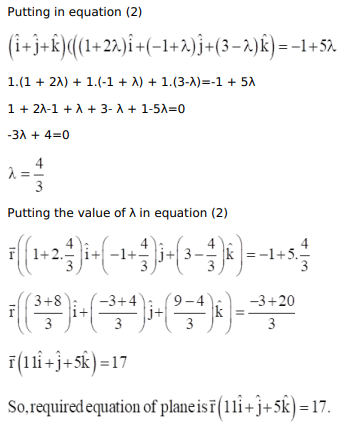# Find the vector equation of the plane through the pointQuestion:

Find the vector equation of the plane through the point $(1,1,1)$, and passing through the intersection of the planes $\vec{r} \cdot(\hat{i}-\hat{j}+3 \hat{k})+1=0$ and $\overrightarrow{\mathrm{r}} .(2 \hat{\mathrm{i}}+\hat{\mathrm{j}}-\hat{\mathrm{k}})-5=0$

Solution: